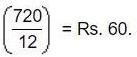Courses

# Test: Profit And Loss- 1

## 10 Questions MCQ Test Quantitative Aptitude for GMAT | Test: Profit And Loss- 1

Description
This mock test of Test: Profit And Loss- 1 for Quant helps you for every Quant entrance exam. This contains 10 Multiple Choice Questions for Quant Test: Profit And Loss- 1 (mcq) to study with solutions a complete question bank. The solved questions answers in this Test: Profit And Loss- 1 quiz give you a good mix of easy questions and tough questions. Quant students definitely take this Test: Profit And Loss- 1 exercise for a better result in the exam. You can find other Test: Profit And Loss- 1 extra questions, long questions & short questions for Quant on EduRev as well by searching above.
QUESTION: 1

### The percentage profit earned by selling an item for Rs. 1920 is equal to the percentage loss incurred by selling the same item for Rs. 1280. At what price should the item be sold to make 25% profit?

Solution: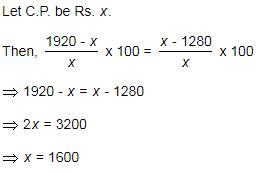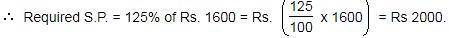QUESTION: 2

### A sells a car to B at 10% loss. If B sells it for Rs. 54000 and gains 20%, the cost price of the car for A was

Solution:

Selling price of the car = Rs.54,000
gain 20%
Friend's cost price of the car = Rs. 54000 x 100/120 = Rs.45,000
Now selling price of the car of the first owner Rs.45000
Loss 10%
Cost price of the car = 45000 x 100/90
= Rs.50,000

QUESTION: 3

### John buys an old scooter for Rs. 4700 and spends Rs. 800 on its repairs. If he sells the scooter for Rs. 5800, what is his gain percent ?

Solution:

Whenever we get this type of question, we should have formula in mind that,
Gain% = (gain/cost) x 100

Cost = 4700 + 800 = Rs. 5500
S.P. = Rs. 5800

Profit = 5800 - 5500 = 300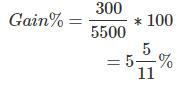QUESTION: 4

Murali purchased 20 dozens of toys at the rate of Rs. 375 per dozen. He sold each one of them at the rate of Rs. 33. Find out his profit percentage.

Solution: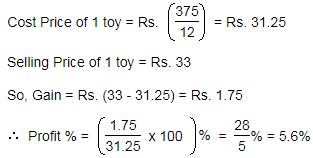QUESTION: 5

A man buys a scooter for Rs. 1400 and sells it at a loss of 15%. What is the selling price of the scooter?

Solution: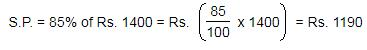QUESTION: 6

In a shop, the profit is 320% of the cost. If the cost increases by 25% but the selling price remains constant, find out approximately what percentage of the selling price is the profit?

Solution:

Let C.P.= Rs. 100. Then, Profit = Rs. 320, S.P. = Rs. 420.

New C.P. = 125% of Rs. 100 = Rs. 125

New S.P. = Rs. 420.

Profit = Rs. (420 - 125) = Rs. 295.QUESTION: 7

The cost price of 20 articles is the same as the selling price of x articles. If the profit is 25%, find out the value of x

Solution:

Let C.P. of each article be Re. 1 C.P. of x articles = Rs. x.

S.P. of x articles = Rs. 20.

Profit = Rs. (20 - x)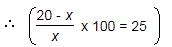⇒ 2000 - 100x = 25x

⇒ 125x = 2000

⇒ x = 16.

QUESTION: 8

A vendor bought bananas at 6 for a rupee. How many for a rupee must he sell to gain 20%?

Solution:

C.P. of 6 toffees = Re. 1

S.P. of 6 toffees = 120% of Re. 1 = Rs.6/5

For Rs.6/5 , toffees sold = 6.

For Re. 1, toffees sold =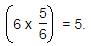QUESTION: 9

An exporter expects a gain of 22.5% on his cost price. If in a week, his sale was of Rs. 392, what was his profit?

Solution: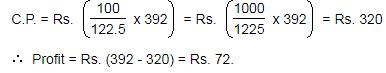QUESTION: 10

On selling 17 balls at Rs. 720, there is a loss equal to the cost price of 5 balls. The cost price of a ball is:

Solution:

(C.P. of 17 balls) - (S.P. of 17 balls) = (C.P of 5 balls)
⇒ C.P of 12 balls = S.P of 17 balls = Rs.720.
⇒ C.P. of 1 ball = Rs.